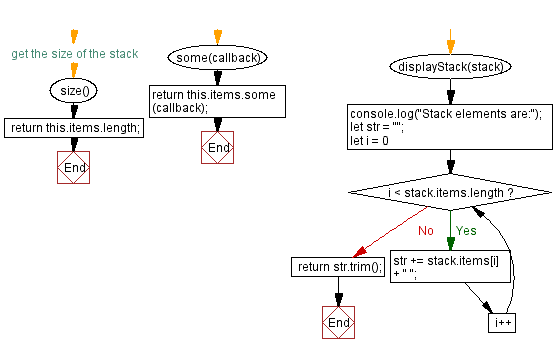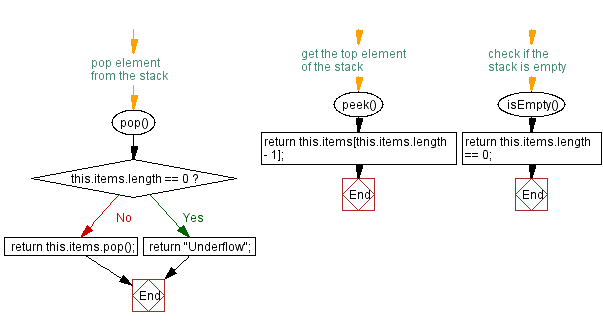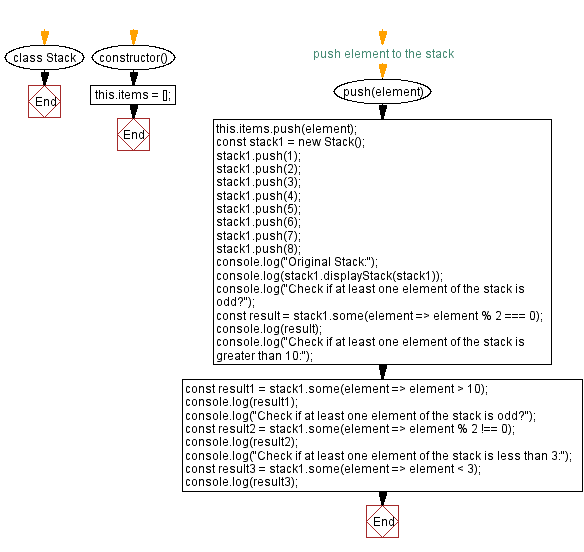# JavaScript Exercises: Verify at least one element satisfy a condition

## JavaScript Stack: Exercise-34 with Solution

Write a JavaScript program that implements a stack and checks if at least one element of the stack satisfies a condition.

Sample Solution:

JavaScript Code:

``````class Stack {
constructor() {
this.items = [];
}
// push element to the stack
push(element) {
this.items.push(element);
}
// pop element from the stack
pop() {
if (this.items.length == 0) {
return "Underflow";
}
return this.items.pop();
}
// get the top element of the stack
peek() {
return this.items[this.items.length - 1];
}
// check if the stack is empty
isEmpty() {
return this.items.length == 0;
}
// get the size of the stack
size() {
return this.items.length;
}
some(callback) {
return this.items.some(callback);
}
displayStack(stack) {
console.log("Stack elements are:");
let str = "";
for (let i = 0; i < stack.items.length; i++)
str += stack.items[i] + " ";
return str.trim();
}
}

const stack1 = new Stack();
stack1.push(1);
stack1.push(2);
stack1.push(3);
stack1.push(4);
stack1.push(5);
stack1.push(6);
stack1.push(7);
stack1.push(8);
console.log("Original Stack:");
console.log(stack1.displayStack(stack1));
console.log("Check if at least one element of the stack is odd?");
const result = stack1.some(element => element % 2 === 0);
console.log(result);
console.log("Check if at least one element of the stack is greater than 10:");
const result1 = stack1.some(element => element > 10);
console.log(result1);
console.log("Check if at least one element of the stack is odd?");
const result2 = stack1.some(element => element % 2 !== 0);
console.log(result2);
console.log("Check if at least one element of the stack is less than 3:");
const result3 = stack1.some(element => element < 3);
console.log(result3);
```
```

Sample Output:

```Original Stack:
Stack elements are:
1 2 3 4 5 6 7 8
Check if at least one element of the stack is odd?
true
Check if at least one element of the stack is greater than 10:
false
Check if at least one element of the stack is odd?
true
Check if at least one element of the stack is less than 3:
true
```

Flowchart:Live Demo:

See the Pen javascript-stack-exercise-34 by w3resource (@w3resource) on CodePen.

Improve this sample solution and post your code through Disqus

Stack Previous: Verify all stack elements satisfy a condition.
Stack Exercises Next: Remove elements from a stack that do not meet a condition.

What is the difficulty level of this exercise?

Test your Programming skills with w3resource's quiz.

﻿